# How to use the formula function of table in WPS Writer

Uploaded time: February 25, 2022 Difficulty: Beginner# How to use the formula function of table in WPS Writer

You know what? In WPS Writer, not only can we perform simple calculations on the table data, but apply the formulas of built-in functions. Here are a few commonly used functions for you to explore.

Take this table as an example.

Assume that we want to count how many  Expense there are in the whole table. We can use the COUNT function to count the number of data in the table.

Tips: The COUNT function in WPS can only be applied in counting number data, while the empty cells, logical values, or text data will be omitted.

Place the cursor in the empty cell at the bottom of the Account number column. In the Table Tools tab, click the Formula button to activate the dialog box.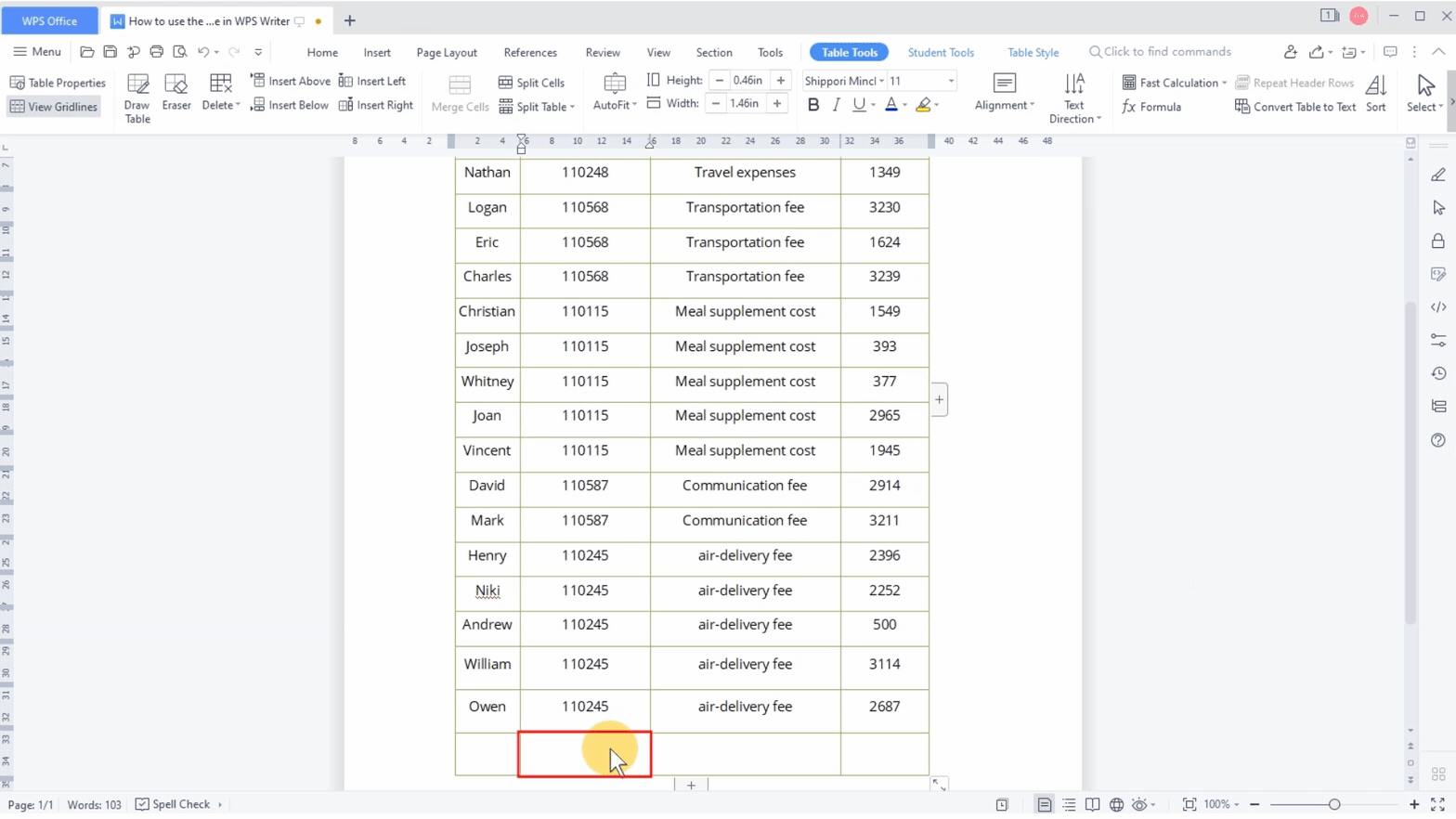In the Paste function area, select the COUNT function. In Table area, select Above and click OK to return the result.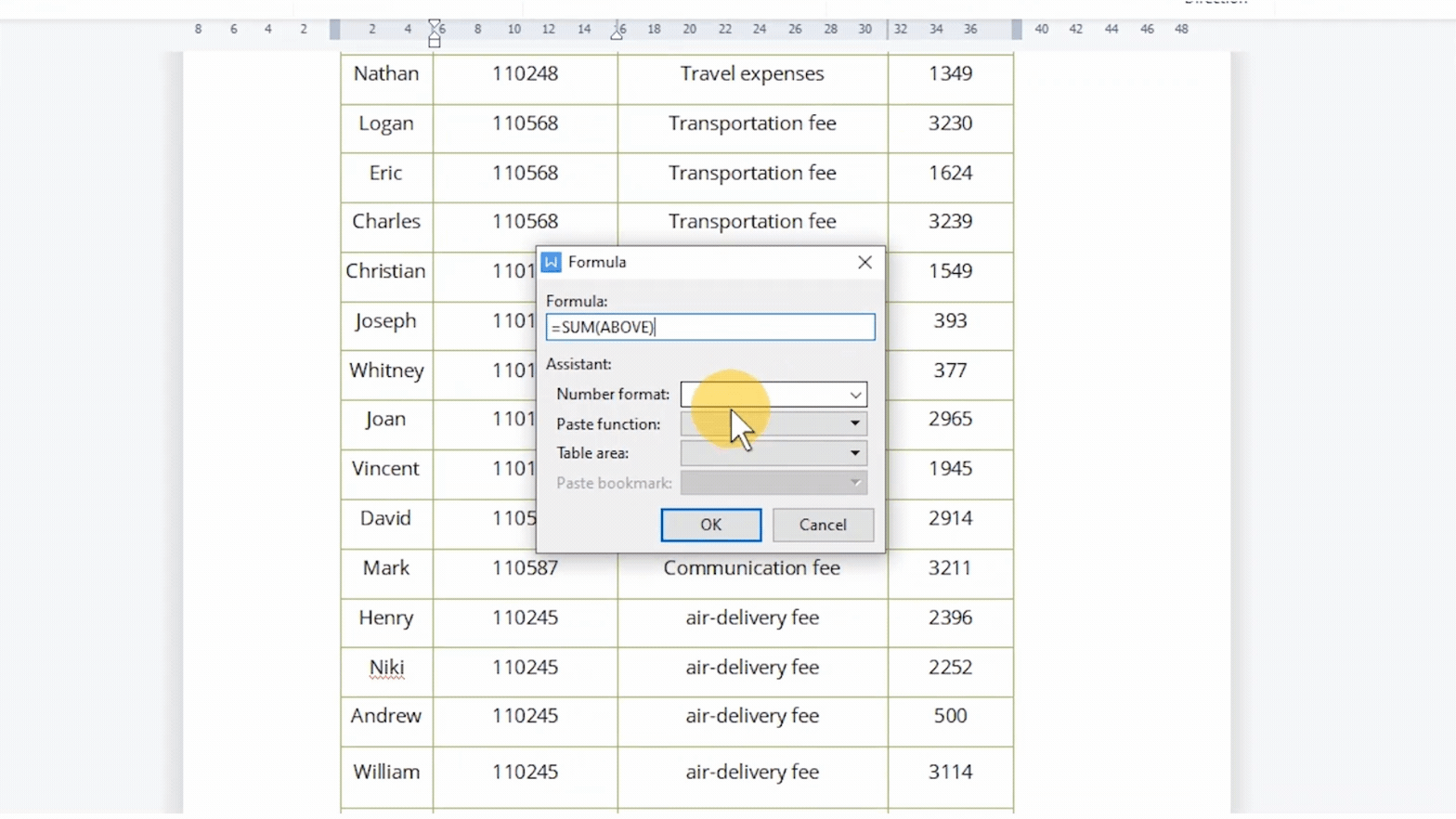Now, suppose that we want to calculate the average of Expense details.

Likewise, click the Formula button under the Table Tools tab.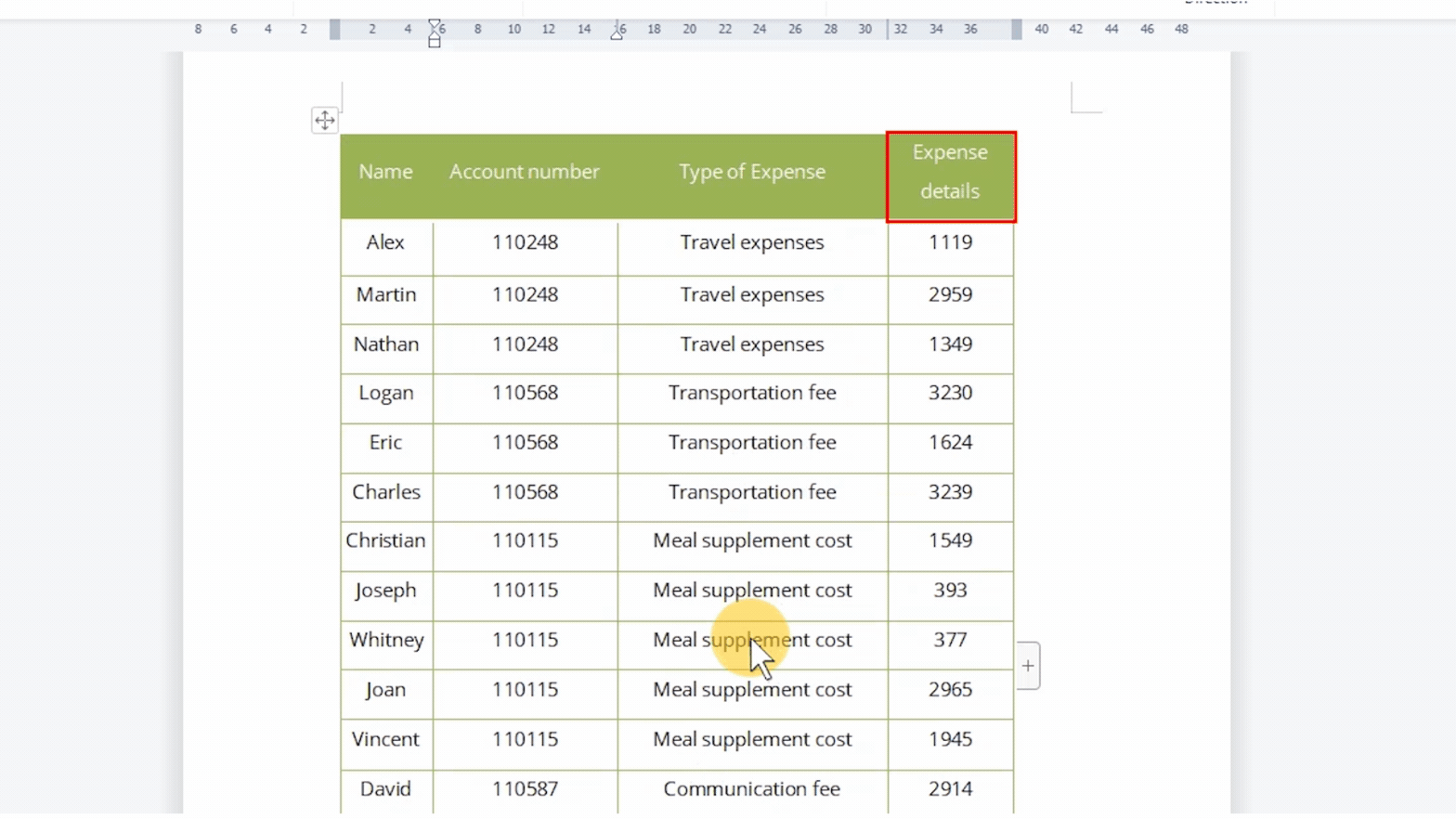In Paste function, choose the AVERAGE function, and set Table area as Above. In the Number format area, we can choose from different formats as needed, such as the thousands separator #,##0.

Click OK to return the calculation result in the empty cell at the bottom of the Expense details column.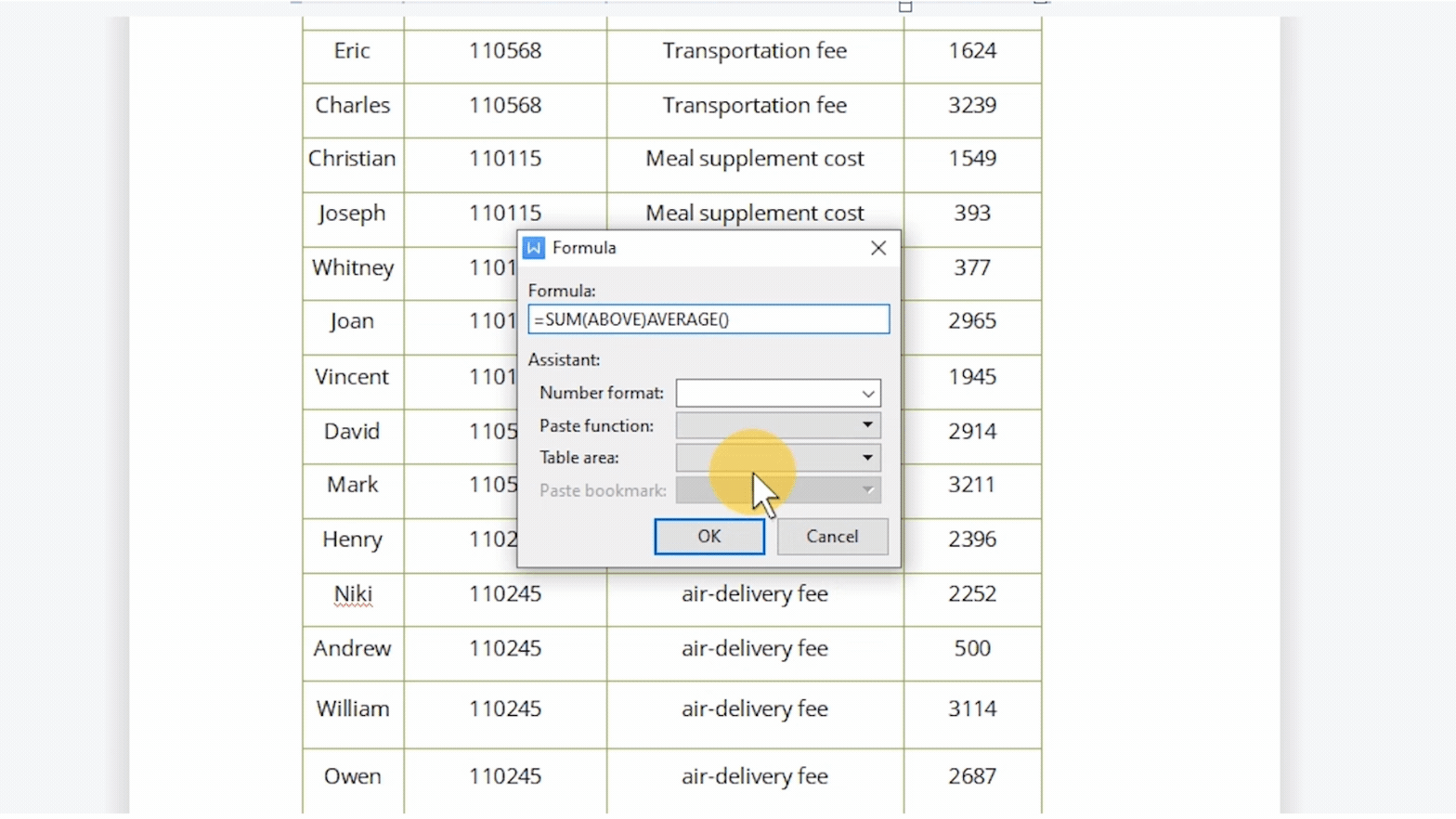Additionally, assume that we want to calculate the average of air-delivery fee, we can select the result we've got before and right-click to choose Edit Field. In the dialog, we can reset the range of values to calculate the average, that is, change Above to D15:D19.

Click OK to return the calculation result in the empty cell at the bottom of the Expense details column.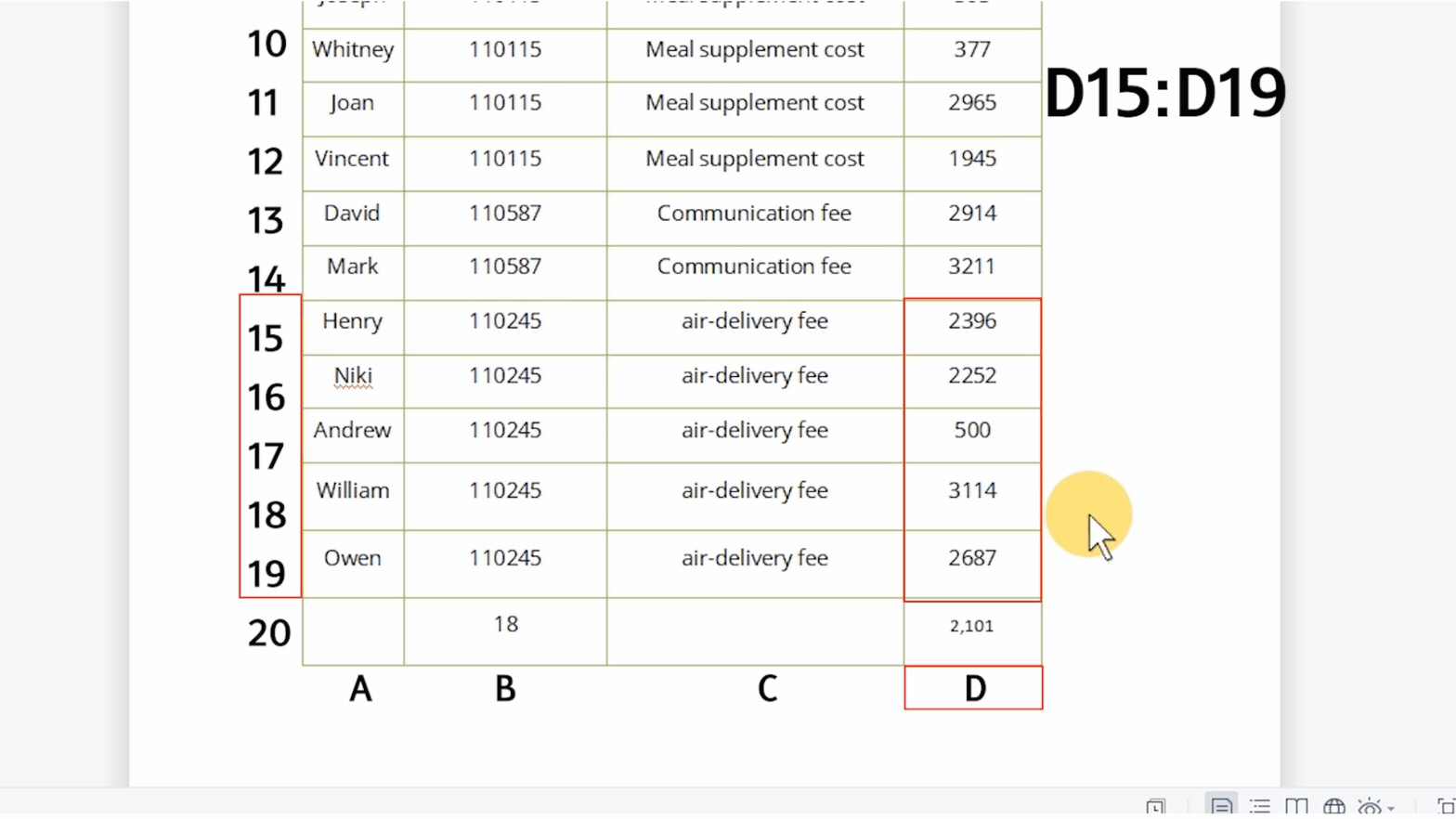Alternatively, we can press the shortcut key Shift+F9 to activate the Toggle Field Codes function. Thus, the calculation result is changed to Field Codes, and the formula can be set directly.

For instance, we can change the calculated range of values back to Above and press Shift+F9 to exit the edit interface. Finally, press F9 to refresh the value.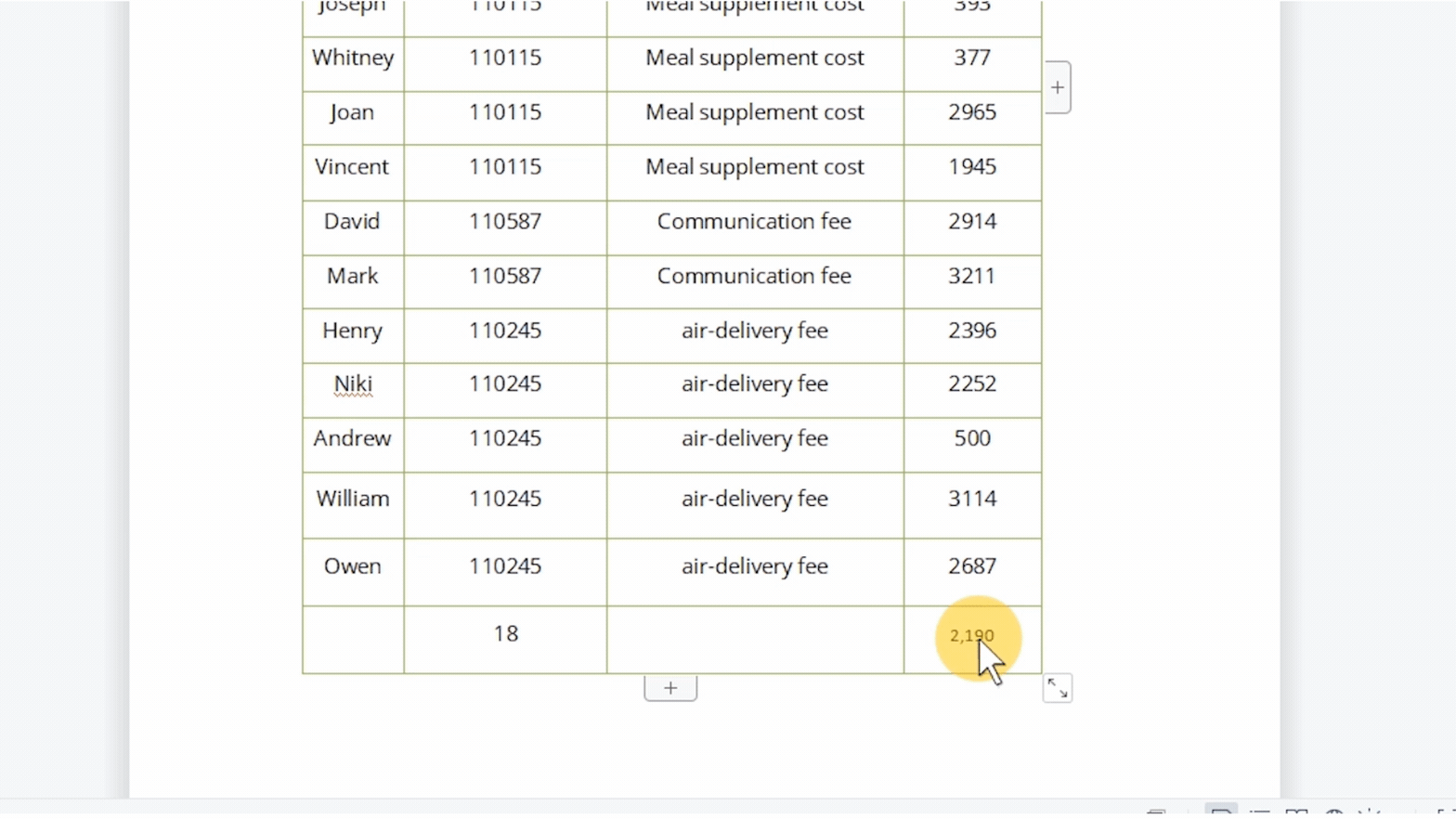In addition to the ones mentioned above, WPS Writer provides plenty of functions for users to apply in the table. Have a try now!